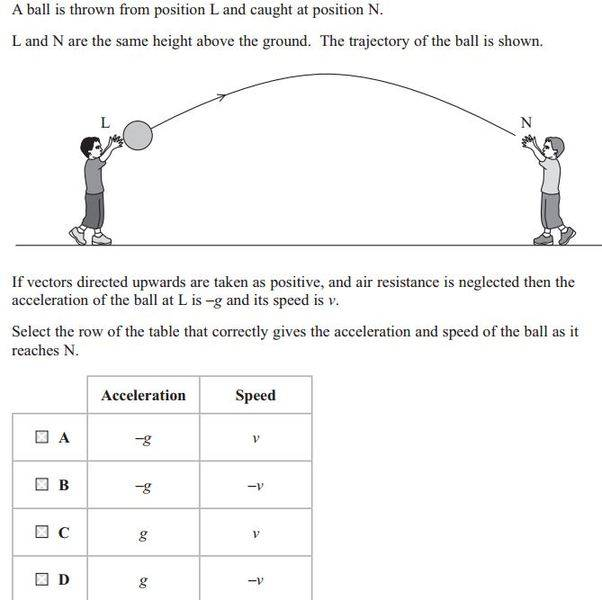# Acceleration and speed

## Homework Statement## The Attempt at a Solution

Since at N the gravity is now heading down towards the person in a positive direction it's acceleration is G, where as velocity goes up then comes down towards the person so it's velocity is now negative, thus -V, but the correct answer is A?? Why do both components stay the same?

gneill
Mentor
Hint: Is speed the same thing as velocity?

Hint: Is speed the same thing as velocity?

Yep, you're right, I confused speed and velocity. So now I understand why speed V is the same.

But for gravity, why has that not changed?

gneill
Mentor
Yep, you're right, I confused speed and velocity. So now I understand why speed V is the same.

But for gravity, why has that not changed?
The Earth didn't move?The direction of the gravitational acceleration vector in the chosen inertial reference frame is independent of the motion of the projectile. Down is still down.